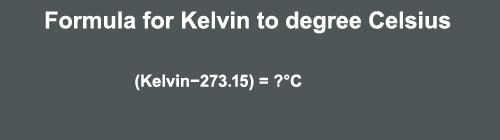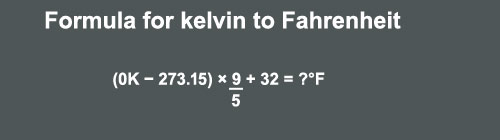# Boiling, Freezing, Melting point of water in kelvin

0
6210

The chemical formula of water is H2O.

The water is a combination of two Hydrogens (H) atoms and one oxygen (O) atom.

Water is the lifeline of all living creatures including humans.

Let us explore the temperature value of water at different parameters such as, in degree Celsius, Fahrenheit, Kelvin.

Degree Celsius to Degree Fahrenheit

The formula for calculating Celsius to Fahrenheit

(Degree Calsius°C × 9/5) + 32 = ?°FFor example

0°C = 32°F

If you want to find 1°C in Fahrenheit, put this value in the formula,

(1°C × 9/5) + 32  = 33.8°F

For finding 10°C

(10°C × 9/5) + 32  = 50°F

and so on…..

Find the value of 50°C in Fahrenheit?

Degree Fahrenheit to Degree Celsius

The formula for calculating Fahrenheit to Celsius

(Degree Fahrenheit°F − 32) × 5/9 = ?°CFor examples

0°F = −17.78°C

(1°F − 32) × 5/9 = −17.22°C

for finding 10°F

(10°F − 32) × 5/9 = −12.22°C

and so on…..

Find the value of 41°in Celsius?

The temperature for the Kelvin scale.

The formula for Degree Celsius to Kelvin

(Degree Celsius°C +273.15) = ?kFor examples

0°C = 273.15 k

(1°C +273.15) = 274.15 k

(10°C +273.15) = 283.15 k

and so on…..

The formula for Kelvin to degree Celsius

(Kelvin−273.15) = ?°CFor examples

0 k = −273.15°C

(1−273.15) = −272.15°C

(10−273.15) = −263.15°C

(300−273.15) = 26.85°C

and so on…..

The formula for calculating Fahrenheit to kelvin

(Degree Fahrenheit°F − 32) × 5/9 + 273.15 = ?kFor examples
0°F = 255.372 k
(1°F − 32) ×5/9 + 273.15 = 255.928 k
(10°F − 32) ×5/9 + 273.15 = 260.928 k
and so on…..

Find the value of 50°F in Kelvin?

The formula to calculate kelvin to Fahrenheit
(0K − 273.15) × 9/5 + 32 = ?°FFor examples
0 k = −459.67 °F
(1k − 273.15) × 9/5 + 32 = −457.87 °F
(10k − 273.15) × 9/5 + 32 = −441.67 °F
and so on…..
Let us find, using these terms,
The boiling point of water in kelvin
The Freezing point of water in kelvin
The melting point of water in kelvin.

## The Boiling point of water in kelvin.

First, we need to find the boiling point of water in degrees Celsius.

The boiling point of water in degree Celsius is 100°C.

Now apply the formula given above of Celsius to the kelvin.

(Degree Calsius°C +273.15) = ?k

(100°C +273.15) = 373.15k

This is the boiling point of water in kelvin.

If you want to explore boiling point such as, in Fahrenheit, you can see the above formulas for that.

### The Melting point of water in kelvin.

The melting point of water in degrees Celsius is 0°C.

Simply apply the formula

(Degree Calsius°C +273.15) = ?k

(0°C +273.15) = 273.15k

You can explore other values by using the above formulas.

#### The Freezing point of water in kelvin.

The freezing point of water in degree Celsius is 0°C.

The formula

(Degree Calsius°C +273.15) = ?k

(0°C +273.15) = 273.15k

These are the values of boiling, freezing and melting point of water in kelvin.

At last…

Save Water Save Earth.